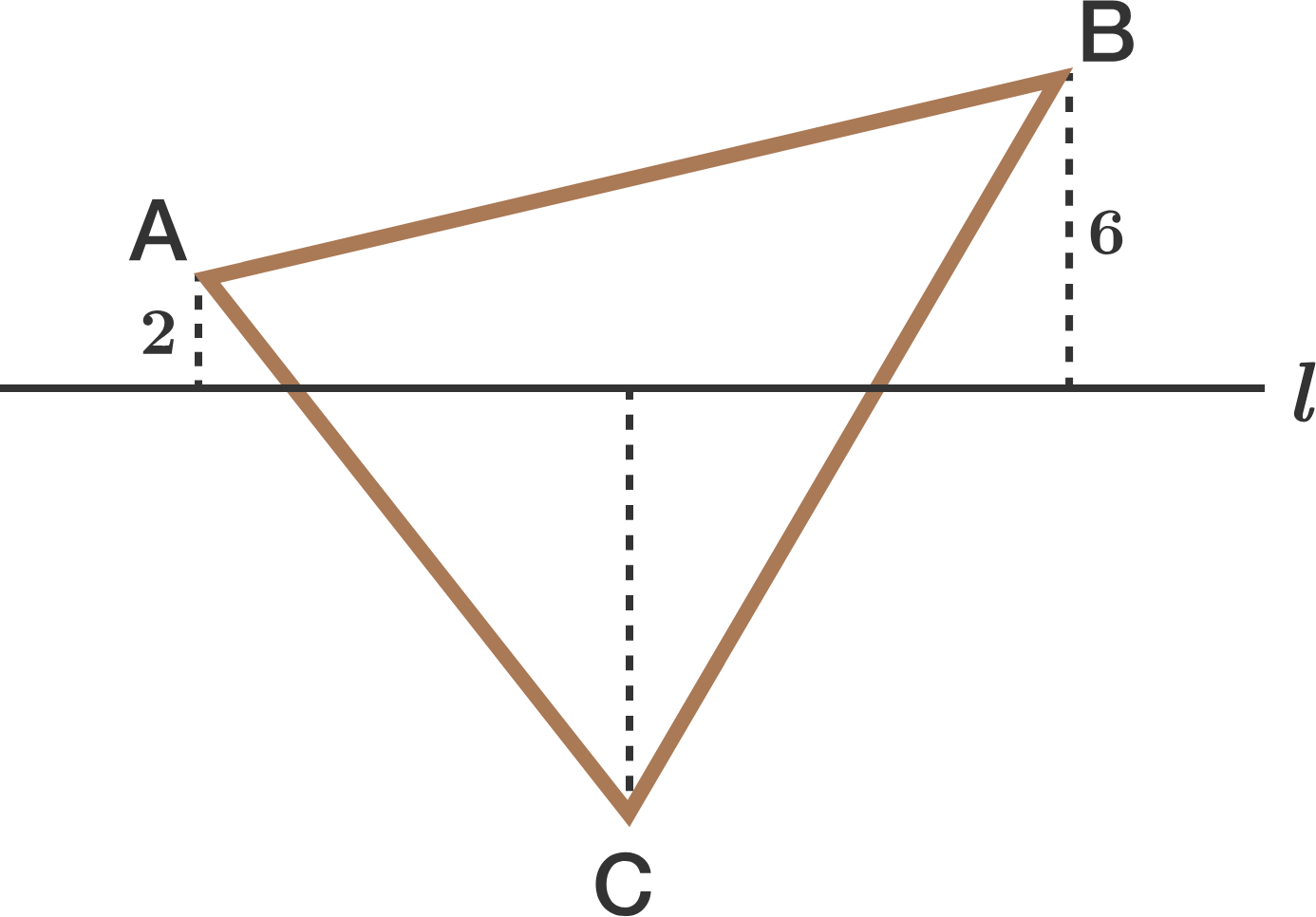# Nasty Triangles 4In the diagram above, line $l$ passes through the centroid of $\triangle ABC.$

If the perpendicular distance between $A$ and line $l$ is 2, and the perpendicular distance between $B$ and line $l$ is 6, then what is the perpendicular distance between $C$ and line $l?$

×